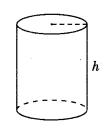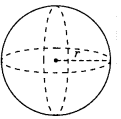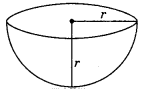On this page, you will find Surface Areas and Volumes Class 9 Notes Maths Chapter 13 Pdf free download. CBSE NCERT Class 9 Maths Notes Chapter 13 Surface Areas and Volumes will seemingly help them to revise the important concepts in less time.

## CBSE Class 9 Maths Chapter 13 Notes Surface Areas and Volumes

### Surface Areas and Volumes Class 9 Notes Understanding the Lesson

Cuboid: With length T, breadth ‘b’ and height ‘h’
(a) Volume = lbh
(b) Total surface area = 2(lb + bh + hl)
(c) Lateral surface area = 2h(l + b) (c)(d) Diagonal = $$\sqrt{l^{2}+b^{2}+h^{2}}$$
(e) Perimeter = 4(l + b + h)

Cube: With side ‘a’
(a) Volume = a3
(b) Total surface area = 6a2
(c) Lateral surface area = 4a2(d) Diagonal = $$\sqrt{3} a$$
(e) Perimeter = 12a

Right circular cylinder: With radius ‘r’ and height ‘h’
(a) Volume = πr2h
(b) Curved surface area = 2πrh(c) Total surface area = 2πr(h + r)

Right circular cone: With radius ‘r’, height ‘h’ and slant height ‘l’
(a) Volume = $$\frac{1}{3}$$ πr2 h or $$\frac{1}{3}$$ x (Area of the base) x height(b) Curved surface area = πrl, where $$l=\sqrt{h^{2}+r^{2}}$$
(c) Total surface area = πr(l + r)

(a) Volume =$$\frac{4}{3}$$ πr3(a) Volume = $$\frac{2}{3}$$πr3